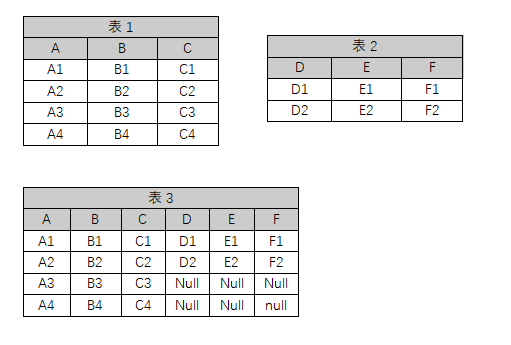SQL 两个无关的查询结果如何合并成多列7个回答

`````` select a.*, b.* from (select ROW_NUMBER() over(order by A) as id, * from table1) a
left join (select ROW_NUMBER() over(order by D) as id, * from table2) b on a.id = b.id
``````

select * from table1
left join table2 on 1=1

mysql亲测可用, 其中 order by ... limit 10, 可以去掉不用的。

select X.A, X.B, X.C, Y.D, Y.E, Y.F from
(Select (@rowNum:=@rowNum+1) as rowNo, A, B, C
From t1, (Select (@rowNum :=0) ) x1
Order by t1.id desc limit 10) X
left join
(Select (@rowNum2:=@rowNum2+1) as rowNo, D, E, F
From t2,
(Select (@rowNum2 :=0) ) y1
Order by t2.id desc limit 10) Y
on X.rowNo = Y.rowNo;

`````` select a.A, a.B, a.C, b.D, b.E, b.F from (select ROW_NUMBER() over(order by A) as id, * from table1) a
left join (select ROW_NUMBER() over(order by D) as id, * from table2) b on a.id = b.id"
``````

`````` using System;
using System.Collections.Generic;
using System.ComponentModel;
using System.Data;
using System.Drawing;
using System.Linq;
using System.Text;
using System.Windows.Forms;
using System.Data.SqlClient;

namespace Q692230
{
public partial class Form1 : Form
{
public Form1()
{
InitializeComponent();
}

private void Form1_Load(object sender, EventArgs e)
{
SqlConnection conn = new SqlConnection("Data Source=.\\SQLEXPRESS;AttachDbFilename=\"C:\\Documents and Settings\\user1\\My Documents\\Q692230DB.mdf\";Integrated Security=True;Connect Timeout=30;User Instance=True");
conn.Open();
string sql =
@"select a.A, a.B, a.C, b.D, b.E, b.F from (select ROW_NUMBER() over(order by A) as id, * from table1) a
left join (select ROW_NUMBER() over(order by D) as id, * from table2) b on a.id = b.id";
SqlCommand cmd = new SqlCommand(sql, conn);
DataSet ds = new DataSet();